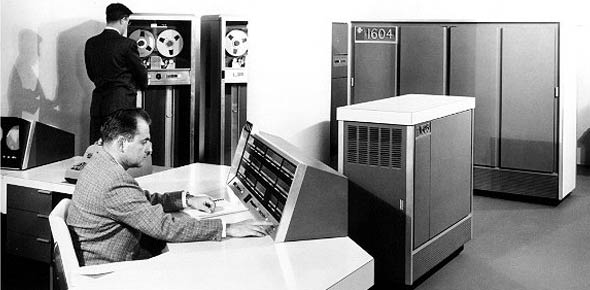# CDC 2e151 Vol 1 Swats Journeyman

29 Questions | Total Attempts: 356SettingsCreate your own QuizThis is a mix of self test and made up questions from the text.

• 1.
X-rays contain what percent of the initial nuclear explosion energy?
• A.

80%

• B.

70%

• C.

60%

• D.

90%

• E.

50%

• 2.
What are the Derived nuclear environments?
• A.

Airblast and shock, thermal radiation, EMP, Atmospheric disturbances, and transient radiation.

• B.

Airblast and shock

• C.

EMP, Atmospheric disturbances, and transient radiation.

• D.

Airblast and shock, and thermal radiation.

• E.

Thermal radiation, EMP, Atmospheric disturbances, and transient radiation.

• 3.
What primary nuclear enviornment elements do we call indirectly ionizing radiation?
• A.

X-ray, Neutrons, and Gamma rays

• B.

Neutrons, and Gamma rays

• C.

Gamma rays and Thermal Radiation

• D.

Thermal Radiation, Neutrons, and Gamma rays

• 4.
What element contains 50% of the derived enviornment energy?
• A.

Airblast and shock

• B.

• C.

Atmospheric disturbances

• D.

• E.

EMP

• 5.
When does the source of radiation occur that causes skin burns, fire, flash blindness, and retinal burns?
• A.

Thermal radiation, second pulse

• B.

Thermal radiation, first pulse

• C.

Transient radiation, second pulse

• D.

Atmospheric disturbances, first pulse

• E.

Transient radiation, first pulse

• 6.
What are the two distinct regions of importance for SREMP?
• A.

• B.

Primary and Derived

• C.

Source and Derived

• D.

• 7.
What is the central issue today concerning EMP?
• A.

Protection

• B.

Avoidance

• C.

Repair

• D.

Shielding

• 8.
What type of EMI occurs when an unwanted carrier frequency follows the normal signal path through the effected receiver?
• A.

Co-Channel

• B.

• C.

Spurious responses

• D.

Spurious emissions

• 9.
What type of intermodulation can have a very large bandwidth and cause more severe interference problems than intermodulation products created in either transmitters or receivers?
• A.

Co-channel

• B.

Brute force

• C.

Spurious response

• D.

Rusty bolt

• 10.
What type of EMI occurs when high level radiated signals penetrate and induce a radio frequency voltage in the internal circuits of a system or equipment?
• A.

Brute force

• B.

Co-channel

• C.

Rusty bolt

• D.

Spurious reponses

• 11.
How soon must you file a report once you confirm an EMI problem exists?
• A.

24 hours

• B.

48 hours

• C.

12 hours

• D.

36 hours

• 12.
What amplitude modulation (AM) receivers are particularly susceptible to power line noise?
• A.

High frequency

• B.

Very-high frequency

• C.

Very-high frequency and High frequency

• D.

Super high frequency

• E.

Very-high frequency, High frequency and Super high frequency

• 13.
What are two types of coaxial transmission lines?
• A.

Rigid and flexible

• B.

Shielded pair and twin lead

• C.

Rigid and parallel two-wire

• D.

Flexible and shielded pair

• 14.
What action takes place when energy is reflected on a transmission line?
• A.

Standing waves form and the line impedance changes

• B.

Standing waves form and causes heat losses

• C.

Standing waves form and cause radiation losses

• D.

Line impedance changes causes heat losses

• 15.
What characteristics are uniform along the length of transmission lines?
• A.

Inductance and Impedance

• B.

Resistance and Inductance

• C.

Capacitance and Impedance

• D.

Resistance, Inductance and Capacitance

• 16.
How much will a horizontal polarized wave rotate the E and H fields?
• A.

90 degrees

• B.

180 degrees

• C.

360 degrees

• D.

45 degrees

• 17.
What is the optical transmission path in a fiber optic cable?
• A.

• B.

Coating

• C.

Core

• D.

Refractive index

• 18.
What part of a fiber optic cable provides the reflective surface that allows light to propagate to the distant end?
• A.

Core

• B.

• C.

Coating

• D.

Normal

• 19.
What part of a fiber optic cable protects it from scratches?
• A.

Core

• B.

• C.

Coating

• D.

Refraction

• 20.
What is the ratio of light's speed in a vacuum to its speed in a medium?
• A.

Reflection

• B.

Refractive index

• C.

Refraction

• D.

Angle of incidence

• 21.
What is the bending of light as it passes through materials of different density?
• A.

Reflection

• B.

Refraction

• C.

Angle of incidence

• D.

Refractive index

• 22.
What is the bouncing of light from an object?
• A.

Refraction

• B.

Reflection

• C.

Refractive index

• D.

Angle of incidence

• 23.
What is the imaginary line perpendicular to the interface of two media?
• A.

Angle of incidence

• B.

Reflection

• C.

Refraction

• D.

Normal

• 24.
What type of fiber optic connector has a conical shape?
• A.

ST connector

• B.

Biconic connector

• C.

SMA connector

• D.

FC connector

• 25.
What type of fiber optic connector uses a push-pull arrangement for mating?
• A.

ST connector

• B.

FC connector

• C.

SMA connector

• D.

SC connector

Related TopicsBack to top
×

Wait!
Here's an interesting quiz for you.